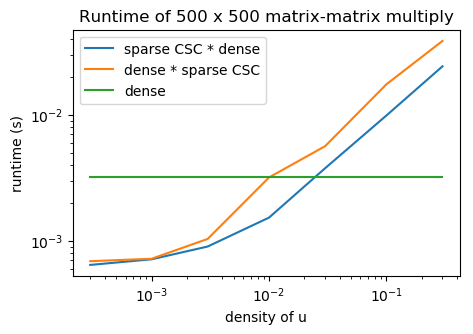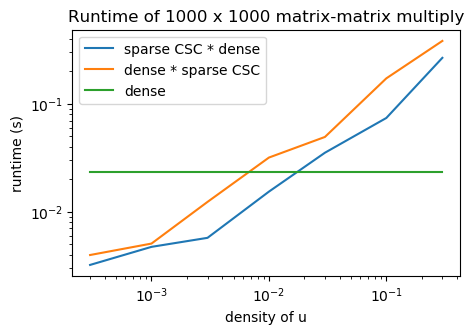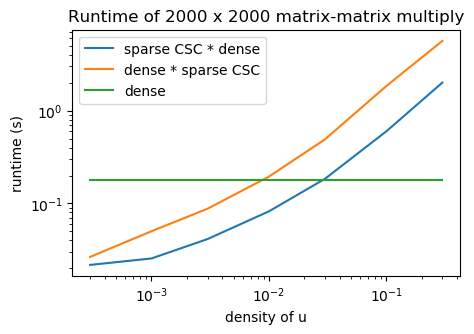## Throughput of Sparse Matrix-Matrix Multiply¶

In :
using PyPlot
using Statistics
using SparseArrays

In :
function sparse_mmpy_test(n::Int64, num_runs::Int64)
x = randn(n,n);
y = randn(n,n);

t_dense = mean([(@elapsed x * y) for i = 1:num_runs]);

dds = [0.0003,0.001,0.003,0.01,0.03,0.1,0.3];
t_sparse_ux = zeros(length(dds));
t_sparse_xu = zeros(length(dds));
for i = 1:length(dds)
dd = dds[i];
u = sprandn(n,n,dd);
t_sparse_ux[i] = mean([(@elapsed u * x) for i = 1:num_runs]);
t_sparse_xu[i] = mean([(@elapsed x * u) for i = 1:num_runs]);
end

figure(figsize=(5.0,3.2));
loglog(dds, t_sparse_ux; label="sparse CSC * dense");
loglog(dds, t_sparse_xu; label="dense * sparse CSC");
loglog(dds, [t_dense for dd in dds]; label="dense");
title("Runtime of $n x$n matrix-matrix multiply");
xlabel("density of u");
ylabel("runtime (s)");
legend();
end

Out:
sparse_mmpy_test (generic function with 1 method)
In :
sparse_mmpy_test(500, 10);In :
sparse_mmpy_test(1000, 10);In :
sparse_mmpy_test(2000, 10);In [ ]:


In [ ]: